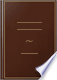بحث صور خرائط YouTube الأخبار Gmail Drive تقويم المزيد »
تسجيل الدخول
 الكتب الكتب 31 - 40 من 194 فيAll the interior angles of any rectilineal figure, together with four right angles,....All the interior angles of any rectilineal figure, together with four right angles, are equal to twice as many right angles as the figure has sides.The Elements of Euclid: viz. the first six books, together with the eleventh ... - الصفحة 28
بواسطة Euclid, Robert Simson - 1835 - عدد الصفحات: 513
عرض كامل - حول هذا الكتاب## Elements of arithmetic, algebra and geometry

George Lees - 1826 - عدد الصفحات: 207
...to all the angles of the figure, together with four right angles ; that is, the angles of the figure are equal to twice as many right angles, as the figure has sides wanting four. PROP. XIII. THEOREM. If two triangles, BAG, EOF, have two angles, BAG, ABC, and a side...
عرض كامل - حول هذا الكتاب## The Elements of Euclid: The Errors by which Theon, Or Others, Have Long ...

Robert Simson - 1827 - عدد الصفحات: 513
...tri• 2 Cor. angles; that is * together with four right angles. There1s, 1. fore all the angles of the figure, together with four right angles, are equal...twice as many right angles as the figure has sides. COR. 2. All the exterior angles of any rectilineal figure are together equal to four right angles....
عرض كامل - حول هذا الكتاب## Elements of Geometry: With Notes

John Radford Young - 1827 - عدد الصفحات: 208
...in each triangle amounts to two right angles, therefore the angles of all the triangles are together equal to twice as many right angles as the figure has sides, that is to say, the sum of the angles of the polygon, together with those about the point within it,...
عرض كامل - حول هذا الكتاب## The First Six Books of the Elements of Euclid, with a Commentary and ...

Euclid, Dionysius Lardner - 1828 - عدد الصفحات: 324
...but the angles at the point F are equal to four right angles (83) ; and therefore the angles of the figure, together with four right angles, are equal...twice as many right angles as the figure has sides. This is the first corollary in the Elements, and the following is the second. (135) COR. 11. — The...
عرض كامل - حول هذا الكتاب## Elements of the Geometry of Planes and Solids: With Four Plates

Ferdinand Rudolph Hassler - 1828 - عدد الصفحات: 159
...angles, as AGD, GDE, and so on, standing in equal segments, are equal to one another; and their sum being equal to twice as many right angles as the figure has sides wanting four: that is, eight right angles, each of these angles of the hexagon is equal eight sixths...
عرض كامل - حول هذا الكتاب## Elements of geometry: Being chiefly a selection from Playfair's geometry

John Playfair - 1829 - عدد الصفحات: 186
...twice — > -fQ as many right angles as there are sides of the figure. But all the interior angles together with four right angles are equal to twice as many right angles as there are sides of the figure (Prop. L). Therefore all the interior and all the exterior angles are...
عرض كامل - حول هذا الكتاب## The London Encyclopaedia: Or, Universal Dictionary of Science ..., المجلد 10

Thomas Curtis - 1829
...twice as 118 119 липу right angles as the figure has sides. Hence the interior angles of the figure are equal to twice as many right angles as the figure has sides wanting four right angles. Cor. 1. All the interior angles of a quadrilateral figure are together equal...
عرض كامل - حول هذا الكتاب## Geometry, Plane, Solid, and Spherical, in Six Books: To which is Added, in ...

Pierce Morton - 1830 - عدد الصفحات: 272
...angle, is equal to two right angles (2.) ; all the interior angles, together with all the exterior angles, are equal to twice as many right angles as the figure has angles. But all the exterior angles are, by the former part of the proposition, equal to four right...
عرض كامل - حول هذا الكتاب## The first six books of the Elements of Euclid: with notes

Euclid, Thomas Elrington - 1833 - عدد الصفحات: 183
...(1): but the angles at the point F are equal to four right angles (2); and therefore the angles of the figure, together with four right angles, are equal...twice as many right angles as the figure has sides. Cor. 7. The external angles of any rectilineal figure are together equal to four right angles. For...
عرض كامل - حول هذا الكتاب## Geometry Without Axioms; Or the First Book of Euclid's Elements. With ...

Thomas Perronet Thompson - 1833 - عدد الصفحات: 150
...isf equal to two right angles ; all the interior together with all the exterior angles of the figure, are equal to twice as many right angles as the figure has sides. But (by Cor. 1 .) all the interior angles are together equal to twice as many right angles as the figure'...
عرض كامل - حول هذا الكتاب